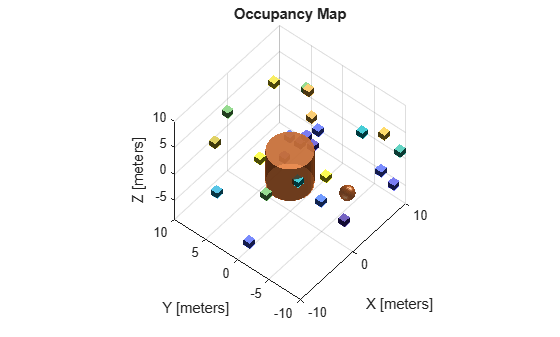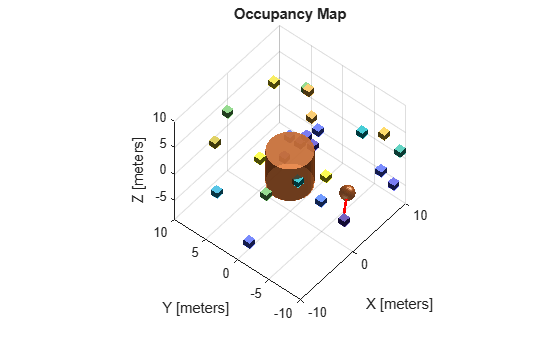# checkMapCollision

Check for collision between 3-D occupancy map and geometry

Since R2022b

## Syntax

``collisionStatus = checkMapCollision(map,geometry)``
``[collisionStatus,details] = checkMapCollision(map,geometry,options)``

## Description

Check for collision between a 3-D occupancy map and a convex collision geometry. The `checkMapCollision` function can search for collisions in two phases: first, a broad-phase search using a broad-phase search using simple bounding volumes, and then by a narrow-phase collision check if the simple volumes collide. For the broad-phase search, the function checks between simple primitives consisting of axially-aligned bounding boxes (AABB). For the narrow-phase, the function checks between occupied cells, represented by a `collisionBox` (Robotics System Toolbox) objects, and the input collision geometry object.

````collisionStatus = checkMapCollision(map,geometry)` checks if a 3-D occupancy map, `map`, and a collision geometry, `geometry`, are in collision.```

example

````[collisionStatus,details] = checkMapCollision(map,geometry,options)` specifies the additional collision-checking options `options`, and returns the collision details `details`.```

## Examples

collapse all

Create a 3-D occupancy map.

`map = occupancyMap3D;`

Specify 25 random coordinates in the occupancy map as occupied.

```rng(0) pt = (rand(25,3)-.5)*20; setOccupancy(map,pt,1);```

Create a collision sphere and a collision cylinder object.

```sphere = collisionSphere(1); cylinder = collisionCylinder(3,6); sphere.Pose = trvec2tform([6.1 -4 -7.5]);```

Visualize the occupancy map and collision geometry in the same figure.

`exampleHelperPlotCylinderAndSphere(map,cylinder,sphere)`Perform only the broad-phase collision check for both the sphere and cylinder by setting the `CheckNarrowPhase` property of an `occupancyMap3DCollisionOptions` object to `false`. Return voxel information and the distance to the nearest occupied voxels.

```bpOpts = occupancyMap3DCollisionOptions(CheckNarrowPhase=false,ReturnDistance=true,ReturnVoxels=true); [bpIsCollidingCylinder,bpResultsCylinder] = checkMapCollision(map,cylinder,bpOpts);```

Check the voxel distances for the collision geometries. Note that, because the cylinder is in collision with voxels, the distance values are `NaN`. Because the sphere is not in collision with any voxels, its distance results are non-`NaN` values.

`bpDistCylinder = bpResultsCylinder.DistanceInfo.Distance`
```bpDistCylinder = NaN ```
`bpWitnessptsCylinder = bpResultsCylinder.DistanceInfo.WitnessPoints`
```bpWitnessptsCylinder = 3×2 NaN NaN NaN NaN NaN NaN ```

Because the cylinder is in collision with the voxels, the distance results contain `NaN` values. Since the sphere is not in collision with the voxels, the distance results consist of non-`NaN` values.

```[bpIsCollidingSphere,bpResultsSphere] = checkMapCollision(map,sphere,bpOpts); bpDistSphere = bpResultsSphere.DistanceInfo.Distance```
```bpDistSphere = 2.3259 ```
`bpWitnessptsSphere = bpResultsSphere.DistanceInfo.WitnessPoints`
```bpWitnessptsSphere = 3×2 3.0000 5.1000 -6.0000 -5.0000 -7.5000 -7.5000 ```

Plot a line between the sphere and the closest voxel to it using its witness points.

```figure exampleHelperPlotCylinderAndSphere(map,cylinder,sphere) hold on plot3(bpWitnessptsSphere(1,:),bpWitnessptsSphere(2,:),bpWitnessptsSphere(3,:),LineWidth=2,Color='r') hold off```Now perform a narrow-phase check, by using an `occupancyMap3DCollisionOptions` object with the `CheckNarrowPhase` property set to `true`.

```npOpts = occupancyMap3DCollisionOptions(CheckNarrowPhase=true,ReturnDistance=true,ReturnVoxels=true); [npIsCollidingSphere,bpResultsSphere] = checkMapCollision(map,sphere,npOpts);```

Return the voxel distance and witness point coordinates for the sphere. The distance and witness points are slightly more accurate this time, because the narrow phase uses the distance between the primitive and the voxel, whereas the broad phase before uses the distance between the axis-aligned bounding box (AABB) of the collision object and the voxel.

`npDist = bpResultsSphere.DistanceInfo.Distance`
```npDist = 2.6892 ```
`npWitnesspts = bpResultsSphere.DistanceInfo.WitnessPoints`
```npWitnesspts = 3×2 3.0000 5.2596 -6.0000 -4.5419 -7.5000 -7.5000 ```

Visualize the occupancy map again and plot line showing the shortest distance between the voxel and sphere. The line between the witness points visually appears accurate after performing the narrow-phase check.

```exampleHelperPlotCylinderAndSphere(map,cylinder,sphere) hold on plot3(npWitnesspts(1,:),npWitnesspts(2,:),npWitnesspts(3,:),LineWidth=2,Color='r') hold off```## Input Arguments

collapse all

3-D occupancy map, specified as an `occupancyMap3D` object.

Example: `occupancyMap3D(10)`

Collision geometry, specified as one of these objects:

• `collisionBox`

• `collisionCapsule`

• `collisionCylinder`

• `collisionMesh`

• `collisionSphere`

Example: `collisionBox(1,1,3)`

Collision-checking options, specified as an `occupancyMap3DCollisionOptions` object.

Example: `occupancyMap3DCollisionOptions(CheckNarrowPhase=false,ReturnDistance=true);`

## Output Arguments

collapse all

Collision status, returned as `0` or `1`. If the geometry intersects with any occupied voxel, `collisionStatus` is `1`. Otherwise, the value is `0`.

Data Types: `double`

Collision details, returned as a structure that can contain up to two fields, `DistanceInfo` and `VoxelInfo`:

• `DistanceInfo` — This field is present when the `ReturnDistance` property of `options` is `true`. The field is a structure that contains these fields.

• `Distance` — If the `NarrowPhase` property of `options` is set to true, this field contains the minimum distance between the collision geometry and the nearest voxel or occupied cell, represented as a `collisionBox` object. If `NarrowPhase` is `false`, then `Distance` is the minimum distance between the AABBs of the collision geometry and the nearest voxel.

For collision geometries that are not in collision, `Distance` is returned as a numeric scalar. For collision geometries that are in collision, `Distance` is returned as `NaN`.

• `WitnessPoints` — Witness points between the collision geometry and the nearest voxel. If the collision geometry is not in collision, `WitnessPoints` is returned as returned as a 3-by-2 matrix, where each column represents the witness points in the form $\left[\begin{array}{c}X\\ Y\\ Z\end{array}\right]$. If the collision geometry is in collision, `WitnessPoints` is returned as `nan(3,2)`.

• `VoxelInfo` — This field is present when the `ReturnVoxels` property of `options` is `true`. The field is a structure that contains these fields.

• `Location` — Center(s) of the colliding voxels. The format of this field depends on the value of the `Exhaustive` property of `options`:

• `true`N-by-3 matrix, where N is the total number of colliding voxels. Each row is a coordinate in the form .

• `false` — Three-element row vector that contains the first collision voxel as a coordinate in the form , or as an empty vector if there is no collision.

• `Size` — Edge lengths of the colliding voxels. The format of this field depends on the value of the `Exhaustive` property of `options`:

• `true`N-element column vector, where N is the total number of colliding voxels. The edge length of each element defines the size of the voxel centered at the corresponding location in `Location`.

• `false` — Edge length of the first colliding voxel, returned as a numeric scalar if there is a collision, or as an empty vector if there is no collision.

## Version History

Introduced in R2022b

expand all